## How to calculate hours worked and minus lunch time in Excel?

As a company employee, we need punch in and punch out every work day, counting the total worked time and minus the lunch time of a day can help to calculate salary according to the time. For example, I have the following daily attendance record which contains log in, log out and lunch start, lunch end times in a worksheet, now, I want to calculate the total hours worked but minus lunch time each day. To finish this job, I can introduce some formulas for you.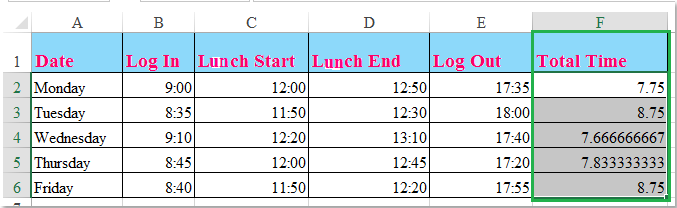Calculate hours worked and minus lunch time with formulas

####Calculate hours worked and minus lunch time with formulas

The following formulas can help you to calculate worked time a day based on the time records.

1. Enter this formula: =SUM((C2-B2)+(E2-D2))*24 into a blank cell beside your time record cells, F2, for instance, see screenshot:

Note: In the above formula: C2 is the lunch start time, B2 is the log in time, E2 indicates the log out time and D2 is the lunch end time. You can change the references based on your need.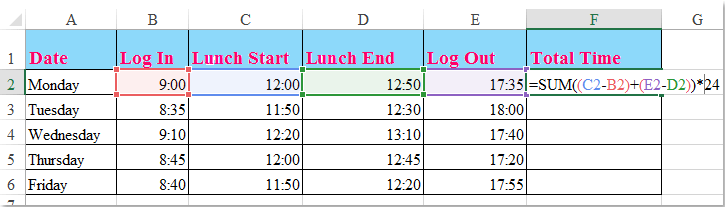2. Then drag the fill handle down to the cells that you want to apply this formula, and all the total hours worked excluding the lunch time are calculated at once. See screenshot: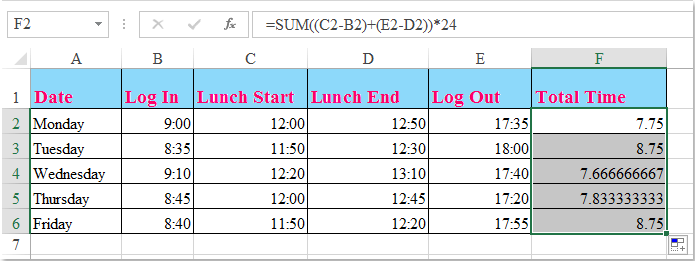Tips: If there are log in, log out time record and fixed lunch time, (such as one hour and thirty minutes), you can apply this simple formula: =D2-B2-C2 to calculate the worked hours a day. See screenshot: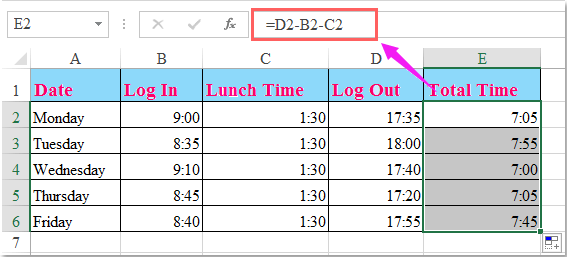### Best Office Productivity Tools

Supports Office/Excel 2007-2021 and 365  |  Available in 44 Languages  |  Easy to Uninstall Completely

 Popular Features: Find/Highlight/Identify Duplicates   |  Delete Blank Rows   |  Combine Columns or Cells without Losing Data   |   Round without Formula ... Super Lookup: Multiple Criteria VLookup  |   Multiple Value VLookup  |   VLookup Across Multiple Sheets   |   Fuzzy Lookup .... Advanced Drop-down List: Quickly Create Drop Down List   |  Dependent Drop Down List   |  Multi-select Drop Down List .... Column Manager: Add a Specific Number of Columns   |   Move Columns   |   Unhide Columns   |   Compare Columns to Select Same & Different Cells ... Featured Features: Grid Focus   |  Design View   |   Big Formula Bar   |  Workbook & Sheet Manager   |  Resource Library (Auto Text)   |  Date Picker   |  Combine Worksheets   |  Encrypt/Decrypt Cells   |  Send Emails by List   |  Super Filter   |   Special Filter (filter bold/italic/strikethrough...) ... Top 15 Toolsets:  12 Text Tools (Add Text, Remove Characters, ...)   |   50+ Chart Types (Gantt Chart, ...)   |   40+ Practical Formulas (Calculate age based on birthday, ...)   |   19 Insertion Tools (Insert QR Code, Insert Picture from Path, ...)   |   12 Conversion Tools (Numbers to Words, Currency Conversion, ...)   |   7 Merge & Split Tools (Advanced Combine Rows, Split Cells, ...)   |   ... and more

Kutools for Excel Boasts Over 300 Features, Ensuring That What You Need Is Just A Click Away...

Supercharge Your Excel Skills: Experience Efficiency Like Never Before with Kutools for Excel  (Full-Featured 30-Day Free Trial)60-Day Unconditional Money-Back Guarantee

#### Office Tab Brings Tabbed interface to Office, and Make Your Work Much Easier

• Enable tabbed editing and reading in Word, Excel, PowerPoint, Publisher, Access, Visio and Project.
• Open and create multiple documents in new tabs of the same window, rather than in new windows.
• Increases your productivity by 50%, and reduces hundreds of mouse clicks for you every day! (Full-Featured 30-Day Free Trial)60-Day Unconditional Money-Back Guarantee

No ratings yet. Be the first to rate!
This comment was minimized by the moderator on the site
Hi there,
I have Cell G9 as Start Time (09:00:00) then F9 as Finish Time (18:00:00) and have a formula for working out the time worked (=IF(F9<E9,F9+1,F9)-E9) but would like to add a formula to calculate If time worked is more that 6 hours, to deduct 00:30:00 minutes for a break and calculate the new time.
This comment was minimized by the moderator on the site
Hello,

=B2-A2-TIME(0,INT((B2-A2)/TIME(6,0,0))*30,0)

B2 is the End time, and A2 is the Start time.
This comment was minimized by the moderator on the site
Добрый день! Подскажите, пожалуйста, у меня есть время прибытия и отбытия, мне нужно ввести формулу сколько на точке пробыл объект и минус 1 час обеда, тоесть если прибыл в 8:00 а отбыл 17:00 итого должно получиться 8 часов
This comment was minimized by the moderator on the site
Hello, Аскар,

B2 is the End time, and A2 is the Start time.
This comment was minimized by the moderator on the site
Ciao, sto cercano una formula o una macro che mi permetta di calcolare le ore di presenza partendo dalle timbrature ma allineate all'orario di lavoro standard.
Per esempio se ho l'orario di lavoro dalle 8:30 alle 12:15 con pausa pranzo e ripresa alle 13.15 fino alle 17:30 (8 ore di lavoro) ma le timbrature sono ad esempio:
Timbratura di Ingresso ore 8:10 con timbratura uscita pausa alle 12:16. timbratura di ingresso dopo la pausa alle 12:50 timbratura di uscita 17:35.

Sicuramente tu potrai aiutarmi. Grazie in anticipo
This comment was minimized by the moderator on the site
Thanks for the info on this website and for your help!!
I've followed the thread and have made my excelsheet. I use this formula =SUM((C5-B5)+(E5-D5)) to calculate the total working hours per day. I don't get the right info if I use =SUM((C5-B5)+(E5-D5))*24 as has been suggested in this thread. I'm using "Time format' instead of general. Even if I choose general I still get an odd number when I type in 8:00AM start and finish 5:30 PM.

Now I want to have the monthly working hours so I use =SUM(L5:L35), but instead of getting the total hours and minutes I get hours/minutes that aren't correct. For example if the total amount is supposed to be 150 hours and 32minutes I get a whole different number.

Note: I have the format in hh:mm

I hope you could help me out.
This comment was minimized by the moderator on the site
Hi, NG,
I'm sorry for that this formula can't help you, You'd better to upload your Excel file here if you don't mind, so that we can check where the problem is?
Thank you!
This comment was minimized by the moderator on the site
Thanks for the reply. How/where do I upload my file? I don't see an attachment possibility.
This comment was minimized by the moderator on the site
Hello, NG,
If there is no "Upload Attachment" box, you should register first, and then the "Upload Attachment" option will be appeared.
To register, please go to the top of the article, and click Resgister button to start.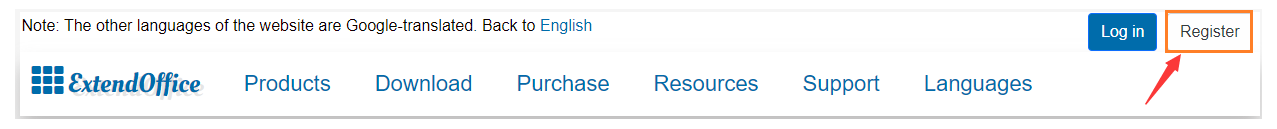I'm sorry for the inconvenience.
This comment was minimized by the moderator on the site
Začátek pracovní doby i konec se vždy liší, jak prosím automaticky odečíst 30 minut bez zadávání začátku a konce přestávky?

Děkuji
This comment was minimized by the moderator on the site

This comment was minimized by the moderator on the site
Very useful! Thanks🤓
This comment was minimized by the moderator on the site
hi 你好，如果餐饮业没有给员工出去用餐一小时（因为公司有包含午餐）那我要怎样计算？正常工作9个小时包含了午餐时间
This comment was minimized by the moderator on the site
hi 你好，如果午餐时间包含在了正常工作时间里，那么只需要用下班时间减去上班时间，就可以得到一天的实际工作时间。希望能帮助到你。
This comment was minimized by the moderator on the site
hello,How can it be worked out the weekly total hours??
This comment was minimized by the moderator on the site
Hello Patricia,The solution I can offer here is to use our formula =SUM((C2-B2)+(E2-D2))*24, then drag the fill handle down to the cells to the rest of cells to calculate the hours per day. Next sum the hours of the five weekdays use the SUM formula =SUM(E2:E6) to get the weekly total hours. Hope this will solve your problem.Sincerely,Mandy
This comment was minimized by the moderator on the site
Is there a way to use that to calculate what you have worked so far during a week and subtract it from your 'scheduled' hours? For instance, if you work a total of 16.47 hours so far for the week and you are scheduled to work 40 total. How would you calculate the amount of time you have left to meet your scheduled hours in excel?
This comment was minimized by the moderator on the site
Hello Mindy,
I think I understand what you mean now. First, in Cell F2, we need to use the formula =SUM((C2-B2)+(E2-D2))*24 to calculate the work hours of Monday. Then drag the fill handle down to cell F6 to calculate the work hours per day in a week. Then we use the formula =40-SUM(F2:F6) to calculate the left work hours in a week. Please see the screenshot, only Monday to Wednesday have records of time, and the working hours of Thursday and Friday are 0. Using formula =40-SUM(F2:F6) returns 16.25. Please have a try. Thanks.
Sincerely,Mandy
This comment was minimized by the moderator on the site
hi, i want to automatically deducted a 1 hour every time i entered the lunch break hours of 11:30 to 12:30,for example i entered the working start time 10:50 and time is 13:10 , and the total hours would be 2 hr. 20minbut i want to subtract the 1 hour , what formula should i use?
This comment was minimized by the moderator on the site
B2 is the End time, and A2 is the Start time.Please try, hope it can help you!
This comment was minimized by the moderator on the site
Thank you! I was having the same problem.  That worked!!
This comment was minimized by the moderator on the site
I am trying to calculate time in, time out, minus 30 min lunch break (fixed each day) I don't track my start lunchtime and end lunchtime since it will always be 30 minutes. Then use the total hours x a given per hour rate.  Is there a way to do this?
I have E7 is clocked in (TIME 13:30 formula) F7 is Clocked out (TIME 13:30 formula), G7 is always 30 minutes lunch break, H7 is total hours worked. each of these is Monday through Sunday  like E7 through E13. H15 is total hours , H16 is rate per hour, H17 is subtotal, H18 is deductions, and J20 is total due. I have everything working except the subtracting of the 30 minute lunch break. That is what I need help with. I need the formula for H7 and how I can then multiply that by \$12.00 per hour. ,
This comment was minimized by the moderator on the site
Hello, Kauffman,
If you just have the clocked in, clocked out and time break, please use this formula to calculate the total time: =D2-B2-C2.
To get the total price per day, please apply this formula: =E2*F2*24. (Note: After inserting this formula, please format the cell as General formatting.)
You can view the below screenshot for the details.
This comment was minimized by the moderator on the site
I just worked at NY Comic Con and was curious how would you calculate the hours if A1 is clocked in (AM/PM), B1 Clocked Out (AM/PM), C1 Break Taken and D1 hours worked. How would the formula look if you place an x in the break taken column, and the break is automatic 1/2 hour?
This comment was minimized by the moderator on the site
I track my start time in A1 and my end time in B1. In C1 is the formula =TEXT(B1-A1,"h") This works, but I can't figure out how to subtract an hour for lunch within this formula. I don't track my start lunchtime and end lunchtime since it will always be 1 hour. Is there a way to do this?
This comment was minimized by the moderator on the site
I am downloading activity reports from Time station (an app that helps monitoring and calculating employees work time and pay.

The in and out are coming one beneath the other and not as you show in your examples (one next to the other).

Is there a way to formulate the table to calculate total daily hours in this layout, considering that I may have multiple entries and exits a day?
This comment was minimized by the moderator on the site
Hello, Avishay,
Sorry, I can't get your point, could you give an example or screenshot here? Thank you!
This comment was minimized by the moderator on the site
@Sheena:

Found something laying around that actually solves your question.

Columns D, E and F are the Start time, End Time and Break.

Have a table somewhere that explains the breaks that have to be calculated:

from till Pause
0:00 6:00 0:00
6:00 9:00 0:30
9:00 10:00 0:45

Then use the following expresssion (note that my reference to the table are on a tab called Anhang):

=IF(AND(D18=ʺʺ,E18=ʺʺ),ʺʺ,(E18+(D18>E18)-D18)-IF((E18+(D18>E18)-D18)>Anhang!\$A\$6,IF(F18>Anhang!\$C\$6,F18,Anhang!\$C\$6),IF((E18+(D18>E18)-D18)<=Anhang!\$B\$4,IF(F18>Anhang!\$C\$4,F18,Anhang!\$C\$4),IF(F18>Anhang!\$C\$5,F18,Anhang!\$C\$5))))
This comment was minimized by the moderator on the site
I need help with a formula. So I'm creating schedules, I have a log in and log out time. If that time is over 6 hours there has to be a 30 minute lunch. I want a formula that will calculate that time worked including a lunch IF it's over 6 hours
This comment was minimized by the moderator on the site
You can use max formula, =MAX(0,CHECK IN-CHECK OUT)-(BREAK-BREAK). Break - break means lunchtime. Example, =MAX(0,M17-H17)-(L17-K17).
This comment was minimized by the moderator on the site
Is there no answer or formula in Excel for this question?
What would the formula be if a minimum of 8.5 hours of work is required but the employee worked 10 hours.
I want a formula ex: 08:50 - 10:00 and then the obvious answer is 01:50 with the option to calculate a weeks overtime or shortfall?
This comment was minimized by the moderator on the site
I too would like the answer to this exact situation!
This comment was minimized by the moderator on the site
Did you get help on this question?
This comment was minimized by the moderator on the site
I would also like the answer to this. Can it be varied? Example: 12 hour shift workers get an hour break, as do 11 and 10 hour shit workers. However, if you work 8 or 9 hours you get 45 minutes deducted, 6 - 7 hours have 30 minutes deducted and 5 hours have 15 minutes deducted. Any hours worked below 5 do not incur a break.
There are no comments posted here yet